Next: CONFIDENCE INTERVALS Up: Resolution Previous: TIME-FREQUENCY-STATISTICAL RESOLUTION

# THE CENTRAL-LIMIT THEOREM

The central-limit theorem of probability and statistics is perhaps the most important theorem in the fields of probability and statistics. A derivation of the central limit theorem explains why the gaussian probability function is so frequently encountered in nature; not just in physics but also in the biological and social sciences. No experimental scientist should be unaware of the basic ideas behind this theorem. Although the result is very deep and is even today the topic of active research, we can get to the basic idea quite easily.

One way to obtain random integers from a known probability function is to write integers on slips of paper and place them in a hat. Draw one slip at a time. After each drawing replace the slip in the hat. The probability of drawing the integer i is given by the ratio ai of the number of slips containing the integer i divided by the total number of slips. Obviously the sum over i of ai must be unity. Another way to get random integers is to throw one of a pair of dice. Then all ai equal zero except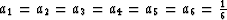.The probability that the integer i will occur on the first drawing and the integer j will occur on the second drawing is ai aj. If you draw two slips or throw a pair of dice, then the probability that the sum of i and j equals k is readily seen to be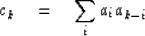(53)
Since this equation is a convolution, we may look into the meaning of the Z transform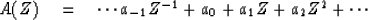(54)
In terms of Z transforms the probability that i plus j equals k is simply the coefficient of Zk in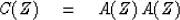(55)
Obviously, if we add n of the random numbers, the probability that the sum of them equals k is given by the coefficient of Zk in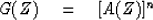(56)
The central-limit theorem of probability says that as n does to infinity the polynomial G(Z) goes to a special form, almost regardless of the specific polynomial A(Z). The specific form is such that a graph of the coefficients of G(Z) comes closer and closer to fitting under the envelope of the bell-shaped gaussian function. Let us see why this happens. Our development will lack a mathematical rigor because the theorem is not always true. There are pathological A functions which do not result in G tending to gaussian. Despite the fact that some of the pathological functions sometimes turn up in applications, we will not take the time here to look at such instances.

Consider the size of A(Z) for real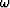.If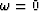, the sum of the terms of A(Z) may be visualized in the complex plane as a sum of vectors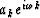all pointing in the positive real direction. If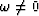the vectors point in different directions. This is shown in Figure 9.

 4-8 Figure 9 The complex numbersadded together.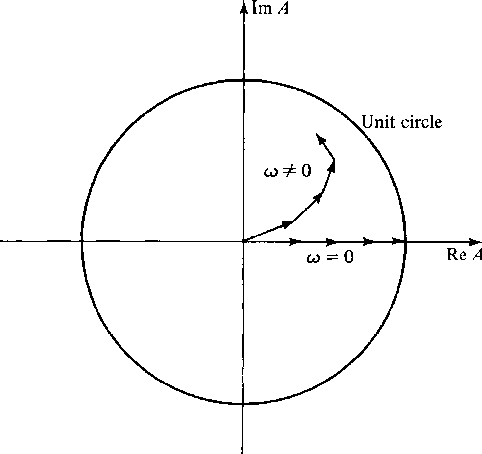In raising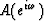to the nth power, the values ofof greatest concern are those nearwhere A is largest--because in any region where A is small An will be extremely small. Nearor Z = 1 we may expand A(Z) in a power series in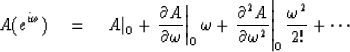(57)
Note that the coefficients of this power series are proportional to the moments mi of the probability function; that is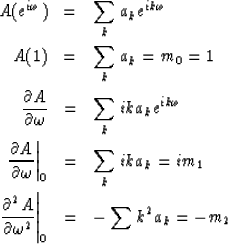(58) (59) (60) (61) (62)
When we raise A(Z) to the nth power we will make the conjecture that only the given first three terms of the power series expansion will be important. (This assumption clearly fails if any of the moments of the probability function are infinite.) Thus, we are saying that as far as G is concerned the only important things about A are its mean value m = m1 and its second moment m2. If this is really so, we may calculate G by replacing A with any function B having the same mean and same second moment as A. We may use the simplest function we can find. A good choice is the so called binomial probability function given by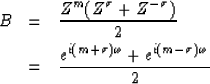(63) (64)
Let us verify its first moment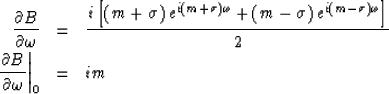(65) (66)
Now let us verify its second moment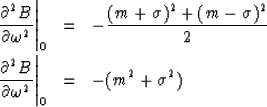(67) (68)
Hence,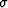should be chosen so that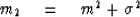(69)
Of course, we cannot expect that m andwill necessarily turn out to be integers; therefore (63) will not necessarily be a Z transform in the usual sense. It does not really matter; we simply interpret (63) as saying:

1.
The probability of drawing the number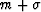is one-half.
2.
The probability of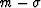is one-half.

3.
The probability of any other number is zero.

Now, raising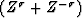to the nth power gives a series in powers of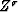whose coefficients are symmetrically distributed about Z to the zero power and whose magnitudes are given by the binomial coefficients. A sketch of the coefficients of B(Z)n is given in Figure 10.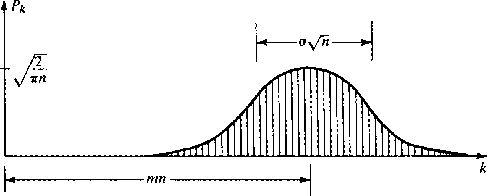4-9
Figure 10
Coefficients of binomial raised to powerWe will now see how, for large n, the binomial coefficients asymptotically approach a gaussian. Approaching this limit is a bit tricky. Obviously, the sum of n random integers will diverge as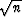.Likewise the coefficients of powers of Z in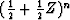individually get smaller while the number of coefficients gets larger. We recall that in time series analysis we used th substitution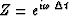.We commonly chose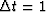,which had the meaning that data points were given at integral points on the time axis. In the present probability theory application of Z transforms, the choicearises from our original statement that the numbers chosen randomly from the slips of paper were integers. Now we wish to add n of these random numbers together; and so, it makes sense to rescale the integers to be integers divided by.Then we can make the substitution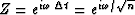.The coefficient of Zk now refers to the probability of drawing the number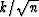.Raising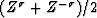to the nth power to find the probability distribution for the sum of n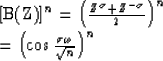Using the first term of the series expansion for cosine we have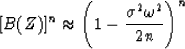Using the well-known fact that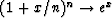, we have for large n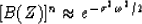(70)

The probability that the number t will result from the sum is now found by inverse Fourier transformation of (70). The Fourier transform of the gaussian (70) may be looked up in a table of integrals. It is found to be the gaussian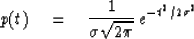Next: CONFIDENCE INTERVALS Up: Resolution Previous: TIME-FREQUENCY-STATISTICAL RESOLUTION
Stanford Exploration Project
10/30/1997